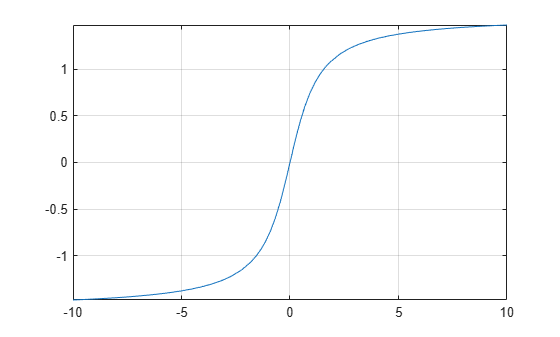# atan

Symbolic inverse tangent

## Syntax

``P = atan(Z)``
``P = atan(Y,X)``

## Description

example

````P = atan(Z)` returns the inverse tangent (arctangent) of the elements of `Z`. All angles are in radians. For real values of `Z`, `atan(Z)` returns values in the interval `[-pi/2,pi/2]`.For complex values of `Z`, `atan(Z)` returns complex values with the real parts in the interval `[-pi/2,pi/2]`. ```
````P = atan(Y,X)` returns the four-quadrant inverse tangent of the elements of `Y` and `X`. This syntax with two input arguments is the same as `atan2(Y,X)`.Symbolic arguments `X` and `Y` are assumed to be real, and `atan(Y,X)` returns values in the interval `[-pi,pi]`.```

## Examples

collapse all

Depending on its arguments, `atan` returns floating-point or exact symbolic results.

Compute the inverse tangent function for these numbers. Because these numbers are not symbolic objects, `atan` returns floating-point results.

`P = atan([-1, -1/3, -1/sqrt(3), 1/2, 1, sqrt(3)])`
```P = 1×6 -0.7854 -0.3218 -0.5236 0.4636 0.7854 1.0472 ```

Compute the inverse tangent function for the numbers converted to symbolic objects. For many symbolic (exact) numbers, `atan` returns unresolved symbolic calls.

`symP = atan(sym([-1, -1/3, -1/sqrt(3), 1/2, 1, sqrt(3)]))`
```symP =  $\left(\begin{array}{cccccc}-\frac{\pi }{4}& -\mathrm{atan}\left(\frac{1}{3}\right)& -\frac{\pi }{6}& \mathrm{atan}\left(\frac{1}{2}\right)& \frac{\pi }{4}& \frac{\pi }{3}\end{array}\right)$```

Use `vpa` to approximate symbolic results with floating-point numbers.

`vpaP = vpa(symP)`
`vpaP = $\left(\begin{array}{cccccc}-0.78539816339744830961566084581988& -0.32175055439664219340140461435866& -0.52359877559829887307710723054658& 0.46364760900080611621425623146121& 0.78539816339744830961566084581988& 1.0471975511965977461542144610932\end{array}\right)$`

Plot the inverse tangent function on the interval from -10 to 10.

```syms x fplot(atan(x),[-10 10]) grid on```Many functions, such as `diff`, `int`, `taylor`, and `rewrite`, can handle expressions containing `atan`.

Find the first and second derivatives of the inverse tangent function.

```syms z D1 = diff(atan(z),z)```
```D1 =  $\frac{1}{{z}^{2}+1}$```
`D2 = diff(atan(z),z,z)`
```D2 =  $-\frac{2 z}{{\left({z}^{2}+1\right)}^{2}}$```

Find the indefinite integral of the inverse tangent function.

`I = int(atan(z),z)`
```I =  $z \mathrm{atan}\left(z\right)-\frac{\mathrm{log}\left({z}^{2}+1\right)}{2}$```

Find the Taylor series expansion of `atan(z)`.

`T = taylor(atan(z),z)`
```T =  $\frac{{z}^{5}}{5}-\frac{{z}^{3}}{3}+z$```

Rewrite the inverse tangent function in terms of the natural logarithm.

`R = rewrite(atan(z),'log')`
```R =  $\frac{\mathrm{log}\left(1-z \mathrm{i}\right) \mathrm{i}}{2}-\frac{\mathrm{log}\left(1+z \mathrm{i}\right) \mathrm{i}}{2}$```

## Input Arguments

collapse all

Tangent of angle, specified as a symbolic number, variable, expression, or function, or as a vector, matrix, or array of symbolic numbers, variables, expressions, or functions.

y-coordinates, specified as a symbolic number, variable, expression, or function, or as a vector, matrix, or array of symbolic numbers, variables, expressions, or functions. All numerical elements of `Y` must be real.

Inputs `Y` and `X` must either be the same size or have sizes that are compatible (for example, `Y` is an `M`-by-`N` matrix and `X` is a scalar or `1`-by-`N` row vector). For more information, see Compatible Array Sizes for Basic Operations.

x-coordinates, specified as a symbolic number, variable, expression, or function, or as a vector, matrix, or array of symbolic numbers, variables, expressions, or functions. All numerical elements of `X` must be real.

Inputs `Y` and `X` must either be the same size or have sizes that are compatible (for example, `Y` is an `M`-by-`N` matrix and `X` is a scalar or `1`-by-`N` row vector). For more information, see Compatible Array Sizes for Basic Operations.

collapse all

### Inverse Tangent

The inverse tangent is defined as

`$\text{atan}\left(Z\right)=\frac{i}{2}\mathrm{log}\left(\frac{1-iZ}{1+iZ}\right).$`

`$\text{atan}\left(Y,X\right)=\text{atan}\left(\frac{Y}{X}\right)+\frac{\pi }{2}\text{sign}\left(Y\right)\left(1-\text{sign}\left(X\right)\right).$`
Results returned by `atan(Y,X)` belong to the closed interval `[-pi,pi]`. Meanwhile, results returned by `atan(Y/X)` belong to the closed interval `[-pi/2,pi/2]`.